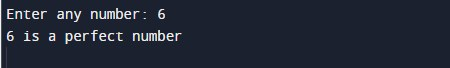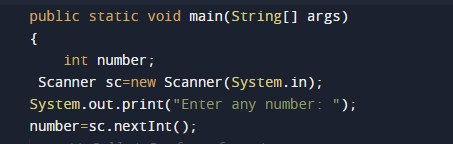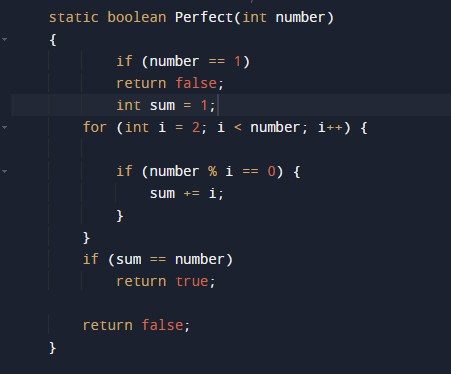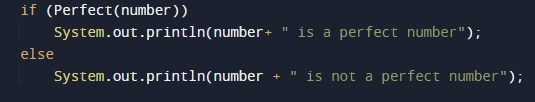# Program to check Perfect Number In Java

In this tutorial you will learn about the Program to check Perfect Number In Java and its application with practical example.

In this tutorial, we will learn to create a Java Program to check Perfect Numbers in Java using Java programming.

## Prerequisites

Before starting with this tutorial we assume that you are best aware of the following Java programming topics:

• Java Operators.
• Basic Input and Output function in Java.
• Class and Object in Java.
• Basic Java programming.
• If-else statements in Java.
• For loop in Java.

## What is Perfect Number.

A number is called Perfect number if Sum of factors (not include the number itself) is equal to the number is called a perfect number.

For example:=>
Number = 6.
Divisors of 6 are 1, 2 and 3.
Sum = 1+2+3 = 6 = 6
⇒ So 6 is a perfect number.

## Program to check Perfect Number in Java

In this program we would find given number is a Perfect number or not .first of we would take a value from user and find the Perfect number.let have a look at the code.

## Output

Perfect  Number.In the above program, we have first declared and initialized a set variables required in the program.

• number= it will hold entered number.
• sum=   it will hold sum of factors.

After that we take a number from user and find given number is a Perfect  number or notafter taking value from user check condition for number is perfect or not.where we first check that number must be grater than one,after that we find the factor of a number and add them and check if sum of factor equals to number then it is a perfect number.if not not a perfect number.

In this tutorial we have learn about the Program to check Perfect Number In Java and its application with practical example. I hope you will like this tutorial.